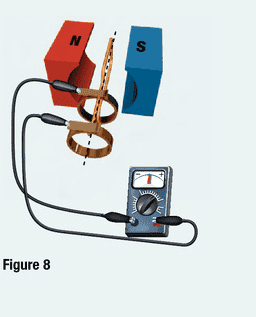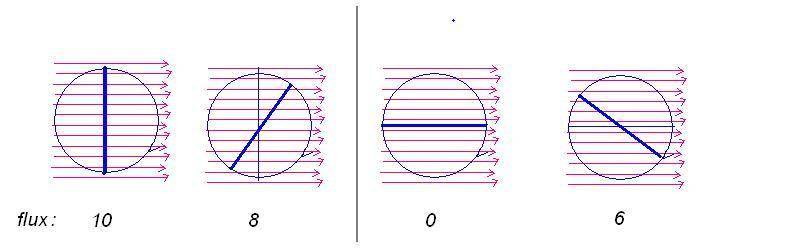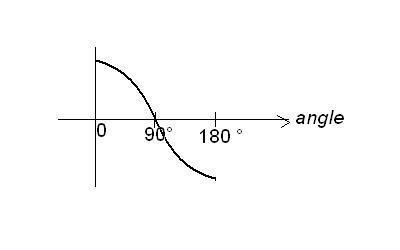# Induced Current in an AC generator

AAAA

## Homework Statement

This is a simple inquiry, based on what my textbook has told me.
"...the amount of induced current also depends on the angle of the conductor in relation to the external magnetic field. The induced current is at a maximum when the plane of the loop is parallel to the external magnetic field. As the loop rotates towards 90° of rotation, the amount of current decreases. Once the loop is completely perpendicular to the magnets (90° of rotation) the current reads zero [in the diagram, the AC generator is connected to a galvanometer]"

The textbook neglects to explain (probably irrelevant, but I'm curious) why the current decreases as the conductor becomes perpendicular to the flux lines of the external magnetic field.N/A

## The Attempt at a Solution

I understand that according to Faraday's Law: A conductor moving relative to a magnet will induce a current in the conductor (because of a change in the magnetic field). And that according to Lenz's Law: If the changing magnetic field induces a current, the current's magnetic field opposes the change that produced it (a magnet moving out of a coil will attract, going into a coil will repel).

I understand that the flux lines of the conductor are going in the same "direction" as the flux lines of the external magnet (If N→S, then conductor has a flux line going clockwise).

So if we need a changing magnetic field, at perpendicular (or as we approach it), does the magnetic field not change? So would the conductor be unaffected by the north and south poles of the external magnet because the push and pull forces have reached an equilibrium? That's the best reasoning I could come up with for this.

Any help is greatly appreciated.

Homework Helper

## Homework Statement

This is a simple inquiry, based on what my textbook has told me.
"...the amount of induced current also depends on the angle of the conductor in relation to the external magnetic field. The induced current is at a maximum when the plane of the loop is parallel to the external magnetic field. As the loop rotates towards 90° of rotation, the amount of current decreases. Once the loop is completely perpendicular to the magnets (90° of rotation) the current reads zero [in the diagram, the AC generator is connected to a galvanometer]"

The textbook neglects to explain (probably irrelevant, but I'm curious) why the current decreases as the conductor becomes perpendicular to the flux lines of the external magnetic field.

N/A

## The Attempt at a Solution

I understand that according to Faraday's Law: A conductor moving relative to a magnet will induce a current in the conductor (because of a change in the magnetic field).

Faraday's Law states that changing magnetic flux across the loop induces electromotive force in the loop. Emf = - dΦ/dt. The minus sign means that the current driven by the Emf produces magnetic field opposing the change of flux.
The loop in the picture rotates in a constant magnetic field. The magnetic flux changes with the orientation of the loop. If it is parallel to the magnetic field, the flux is zero, if it is perpendicular to the field, the flux is maximum. The flux varies with the angle of rotation as Φ0sin(α). At what positions is the derivative of the flux biggest, and where is it zero?

Staff Emeritus
The textbook neglects to explain (probably irrelevant, but I'm curious) why the current decreases as the conductor becomes perpendicular to the flux lines of the external magnetic field.
Because in that position, a few degrees of rotation of the loop doesn't cause much change in the number of flux lines threading the loop. Flux through the loop is approximately fixed as the loop rotates in that region, so dΦ/dt is close to zero.

Contrast this with the rapidly changing flux when the loop is parallel to the magnetic field.

AAAA
We never did any math with EM fields, but let me see if I can understand what you guys wrote:
If emf = - dΦ/dt
And d theta is sin of the angle of rotation→ then -sin(90)/100ms (I've never used this equation before, so I'm not sure if I'm using it right)→ then your emf = -0.00893996664 s/m.
If the angle of rotation is 0, then it's 0 s/m. So @ perpendicular→ the angle of rotation is 0? In my book there is a graph of a sine wave with current in the y axis, and degrees of rotation on the x axis. @ 90 degrees of rotation→ the current is 0. At 0degrees→ the current is max. But I guess that's relative to your starting position.

a few degrees of rotation of the loop doesn't cause much change in the number of flux lines threading the loop
I think this makes sense to me, you're not crossing many of the flux lines created by the external magnet at the very top, so less change. That makes sense. Is that what you mean?

I'm not sure about some of what you guys are talking about, we're doing very basic stuff in our class. Could we go a bit higher level? :D

Last edited:
Homework Helper
See the figure. The red lines represent the magnetic field lines. The flux is the number of the lines crossing the cross section of the loop (drawn by blue)
The flux is shown in each position of the loop. It is maximum when the loop is perpendicular to the field lines, that is, vertical . Turning from the vertical position with some small angle, the flux decreases from 10 to 8.
In the horizontal position, the flux is zero, and turning the loop with the same angle as before, the flux increases by 6.•AAAA
AAAA
I understand now, from your diagram, that the flux changes as the cross-section of the loop covers fewer lines or more magnetic field lines. What I don't understand is how that affects the amount of current. Why isn't a loop parallel to the external field lines creating a current of 0, if it's not crossing any of the flux lines? How does that cause more of a change in the magnetic field than if you were crossing over 10 field lines→which creates no current?

Staff Emeritus
induced current is not proportional to Φ

induced current is proportional to dΦ/dt

you have to look at how the enclosed flux is changing with time

AAAA
you have to look at how the enclosed flux is changing with time
I believe this is what ehild has drawn above.

If so, what I don't understand is how having a greater flux leads to a lesser current.

Staff Emeritus
If so, what I don't understand is how having a greater flux leads to a lesser current.
It doesn't.

Greatest flux happens (from the geometry of the situation) to coincide with the moment when dΦ/dt is at zero, and it's this zero value of dΦ/dt which is causing the zero current. It is this which ehild is showing in those diagrams.

Homework Helper
The current is proportional with the change of the flux. Turning with a small angle from the vertical position of the loop causes small change of the flux and the flux changes most around the horizontal position of the loop.

AAAA
The current is proportional with the change of the flux. Turning with a small angle from the vertical position of the loop causes small change of the flux and the flux changes most around the horizontal position of the loop.
How is it that in the vertical position, less of the flux is changed, when you are crossing over more flux lines(10, in that diagram)? This, in comparison to crossing over say 6 in the fourth part of the diagram.

That's what's confusing me.

Or is this some lower level detail that is beyond the scope of my knowledge?

Homework Helper
In the first picture, the loop is vertical and 10 lines crosses it. In the second one, the loop is turned by some small angle and you count 8 lines crossing it. The change of flux is -2.
In the third picture, the loop is horizontal initially and crosses no lines, the flux is zero. In the fourth picture, the loop is turned by the same angle as previously, and crosses 6 lines. The change of flux by turning the he loop is 6.
Mathematically, the flux is proportional to the area perpendicular to the magnetic field, which is the area of the loop multiplied by cos(α), the cosine of the angle, enclosed by the field lines and the normal of the loop. The cosine is zero at 90° angle, (horizontal loop) and changes fastest when the angle is changed. When the loop is vertical, the angle is zero, the cosine is 1, and its change is the slowest.